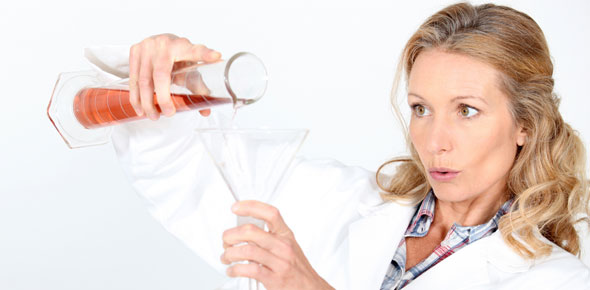# Chemical Equations Assessment Noel Pd 2

21 Questions | Attempts: 52
ShareSettings• 1.
Which equation listed below is an example of a synthesis reaction?
• A.

A + B yields AB

• B.

AB yields A + B

• C.

AB + C yields AC + B

• D.

AB + CD yields AC + BD

• 2.
Which equation listed below is an example of a decomposition reaction?
• A.

A + B yields AB

• B.

AB yields A + B

• C.

AB + C yields AC + B

• D.

AB + CD yields AC + BD

• 3.
Which equation listed below is an example of a single replacement reaction?
• A.

A + B yields AB

• B.

AB yields A + B

• C.

AB + C yields AC + B

• D.

AB + CD yields AC + BD

• 4.
Which equation listed below is an example of a double replacement reaction?
• A.

A + B yields AB

• B.

AB yields A + B

• C.

AB + C yields AC + B

• D.

AB + CD yields AC + BD

• 5.
The _____________ are on the left side of the yield sign in a chemical reaction and are described as the "ingredients" in a chemical reaction.
• A.

Reactants

• B.

Products

• 6.
The _____________ are on the right side of the yield sign in a chemical reaction and are described as what is produced  in a chemical reaction.
• A.

Reactants

• B.

Products

• 7.
The arrow in a chemical reaction means all of the following except
• A.

Yields

• B.

Produces

• C.

Decomposes

• D.

Attaches to

• 8.
The Law of Conservation of Matter states
• A.

Matter cannot be created or destroyed in a chemical reaction

• B.

Matter can only be created, never destroyed in a chemical reaction

• C.

Matter can only be destroyed, never created in a chemical reaction

• D.

Matter can be both created and destroyed in a chemical reaction

• 9.
Painting a pencil red  is a chemical change.
• A.

True

• B.

False

• 10.
Check ALL of the properties listed below that are chemical properties.
• A.

Flammability

• B.

Combustability

• C.

Flexible

• D.

Affected by light

• E.

Ability to rust

• 11.
Select ALL the answers listed below that are examples of a physical change.
• A.

Color change

• B.

A match burning

• C.

Sodium reacting with water

• D.

Water changing to steam

• E.

Wood being shreded

• 12.
Select ALL the choices below that are examples of a chemical change.
• A.

Iron rusting

• B.

Color change as an indicator

• C.

Baking soda fizzing/bubbling when mixed with vinegar

• D.

Water turning into ice

• E.

Breaking a pencil

• 13.
Select ALL the choices below that are examples of a physical property.
• A.

Bitter taste

• B.

Odor

• C.

Ability to rust

• D.

Melting point

• E.

Reacts with an acid

• 14.
Which of the following best describes something that has mass and takes up space?
• A.

Chemistry

• B.

Matter

• C.

Chemical change

• 15.
The study of matter and how it changes is best described as
• A.

Chemistry

• B.

A property of matter

• C.

A chemical change

• D.

Matter

• 16.
A change in matter that results in something new is called
• A.

Chemistry

• B.

Physical change

• C.

Chemical change

• D.

Matter

• 17.
A characteristic that can be observed without changing the substance is called a ______________.
• A.

Physical property

• B.

Physical change

• C.

Chemical property

• D.

Chemical change

• 18.
A(n) _____________ chemical reaction occurs when heat is absorbed into a chemical reaction.
• A.

Natural

• B.

Synthetic

• C.

Endothermic

• D.

Exothermic

• 19.
A(n) _____________ chemical reaction occurs when heat is released from a chemical reaction.
• A.

Natural

• B.

Synthetic

• C.

Endothermic

• D.

Exothermic

• 20.
Select ALL the options listed below that are a sign of a chemical reaction.
• A.

Temperature increase

• B.

Temperature decrease

• C.

Light is produced

• D.

Change in shape

• E.

Food coloring in water

• F.

Fizzing/bubbling

• 21.
A characteristic of a substance that describes its ability to change into something new is called a(n)
• A.

Physical change

• B.

Chemical property

• C.

Chemical change

• D.

Physical property

## Related TopicsBack to top
×

Wait!
Here's an interesting quiz for you.